# Mr G S LAWANIA

•### Eighth Root of a number

Activity

Mr G S LAWANIA

•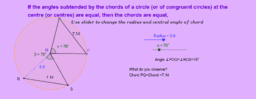### If the angles subtended by the chords of a circle (or of congruent circles) at the centre (or centres) are equal, then the chords are equal,

Activity

Mr G S LAWANIA

•### There is one and only one circle passing through three given non-collinear points,

Activity

Mr G S LAWANIA

•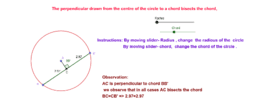### The perpendicular drawn from the centre of the circle to a chord bisects the chord,

Activity

Mr G S LAWANIA

•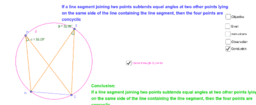### If a line segment joining two points subtends equal angles at two other points lying on the same side of the line containing the line segment, then the four points are concyclic

Activity

Mr G S LAWANIA

•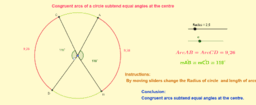### Congruent arcs of a circle subtend equal angles at the centre

Activity

Mr G S LAWANIA

•### Equal chords of a circle are equidistant from the centre.

Activity

Mr G S LAWANIA

•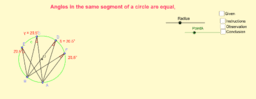### Angles in the same segment of a circle are equal

Activity

Mr G S LAWANIA

•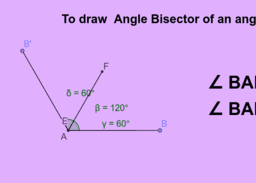### Angle Bisector

Activity

Mr G S LAWANIA

•### Rhombus

Activity

Mr G S LAWANIA

•### Line Segment, Ray and Line

Activity

Mr G S LAWANIA

•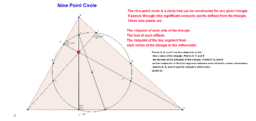### Nine Point Circle

Activity

Mr G S LAWANIA

•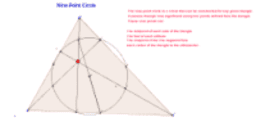### Nine Point Circle

Activity

Mr G S LAWANIA

•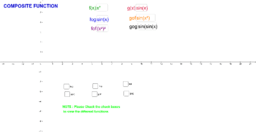### Composite Function

Activity

Mr G S LAWANIA

•### Value of Trigonometric Ratios in Different Quadrants

Activity

Mr G S LAWANIA

•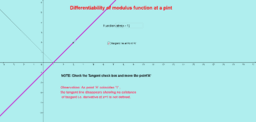### Differentiability of Modulus Function

Activity

Mr G S LAWANIA

•### Arithmetic Progression Practice

Activity

Mr G S LAWANIA

•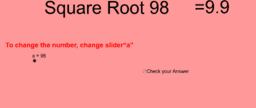### To find Square Root of a Positive Number

Activity

Mr G S LAWANIA

•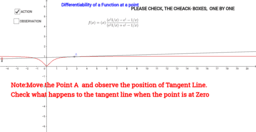### Differentiability of a function at a given point

Activity

Mr G S LAWANIA

•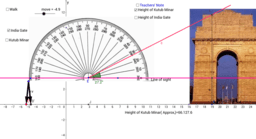### Application of Trigonometry ( Heights and Distances )

Activity

Mr G S LAWANIA

•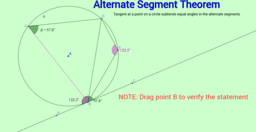### Alternate Segment Theorem

Activity

Mr G S LAWANIA

•### Volume and Surface Area of Cone

Activity

Mr G S LAWANIA

•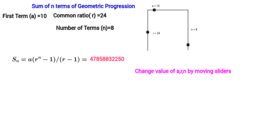### Geometric Progression_Sum of 'n' Terms

Activity

Mr G S LAWANIA

•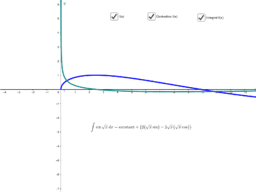### Concept of Derivative and Integral

Activity

Mr G S LAWANIA